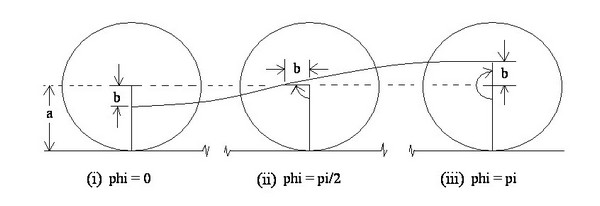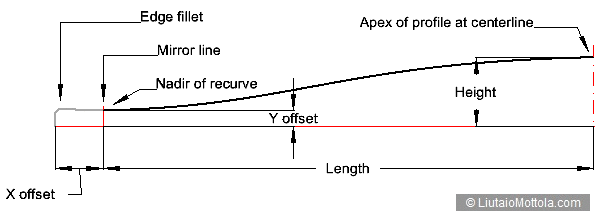# Calculating Curtate Cycloid Points

Curtate cycloids make nicely shaped curves which are often used as arching profiles for arched plate instruments such as violins and archtop guitars. Generating these curves for use as arching profiles is somewhat problematic because the formulae used to specify points on the curve are parametric functions of phase angle. It would be much more convenient to use a Cartesian function, but such a thing doesn't exist. This page contains a Javascript calculator which implements this functionality. It can be used to generate a series of points with fixed x or fixed y interval. These point series can then be used for analysis and comparison, or to generate contour lines.

Initial appearance: November 7, 2010
Last updated: July 31, 2020

## Curtate Cycloids

A curtate cycloid curve is generated as shown in the following diagram, drawn by David Cohen of Cohen Musical Instruments and which appeared in his American Lutherie article "Curtate Cycloid Arching"1:Violin makers and guitarmakers first became interested in these curves for purposes of arching profiles of carved plate instruments following publication of an article by Quentin Playfair2 in which he compared these curves to arching profiles of golden age violins. They don't really model the arching curves of violins well at all3 but they are nice looking curves and are readily rendered (curves that more realistically model those of Cremonese violin arching profiles can be found here).

The parametric equations for calculating locations of points on a curtate cycloid curve are:

x = aφ - b sin φ
y = a - b cos φ

where:

a is the radius of the circle;
φ is the phase, 0 to π;
b is some displacement from the center of the circle;

As you can see, the equations yield coordinate values as functions of phase φ. For purposes of generating graphs or graphic curves, it is highly desirable to be able to generate points using a fixed x interval. And for purposes of generating a series of contour lines approximating an arched surface or for generating instructions to a CNC machine for cutting an arched plate, it would be convenient to have a series of points that used a fixed y interval (contour interval).

## Javascript Calculator

The equations presented do not provide this unfortunately. I needed this functionality to generate some curtate cycloid curves for research and so developed the following Javascript calculator for that purpose. The calculator generates a list of points for a half curtate cycloid curve with either a fixed x interval or a fixed y interval. The calculator takes the following arguments:

### Length of half cycloid

The length of the half cycloid curve;

### Total height

The total height of the half cycloid curve;

### X offset (indent)

The script assumes that you are using the output to generate curves for the contour of a plate. This value indicates how far in from the edge of the plate the curve should start. It is added to each x coordinate value generated by the script;

### Y offset (thickness)

This value is equivalent to the thickness of the plate at the start of the curve. It is added to each y coordinate value generated by the script. This value must be less than the total height;

### Number of points

The number of data points you want generated;

Here is how these parameters relate to a typical transverse arching profile:The generated curve is shown in black in the above drawing. It should be obvious how to mirror a piece of the start of the curve to form the recurve area, and how to mirror the whole thing to get a profile for the right side of the plate too.

Note that the x or y interval is specified implicitly. When requested to generate a list of points for a half curtate cycloid curve with a fixed x interval, the calculator uses (the length - the x offset) divided by (the number of points - 1) as the x interval. When requested to generate a list of points for a half curtate cycloid curve with a fixed y interval, the calculator uses (the total height - the Y offset) divided by (the number of points - 1) as the y interval.

You can specify the format you want for the output. The calculator will generate a tab delineated .csv file for use by a spreadsheet, a .dxf file containing a polyline representing the specified curve in default units for use by CAD drawing software, or an .svg file containing a polyline representing the specified curve in default units for use by artistic drawing software. The calculator will also generate a coordinate list output in a popup window. If you specify this be sure to allow popups for this website. The coordinate list is output as a list suitable for copying to the clipboard and then being pasted into a spreadsheet or other program for further use.

Here's the calculator which generates a list of points for a half curtate cycloid curve:

### Calculating Points on a Curtate Cycloid Curve

 Which coordinate interval will be fixed? Fixed x interval Fixed y interval Length of half cycloid: Total height: X offset (indent): Y offset (thickness): Number of points: Where do you want the output to go? Download as .csv file Download as .dxf file containing open polyline Download as .svg file containing open polyline Display results in a new window (Turn off popup blocker.) Javascript must be enabled in your browser to use the calculator. Status of calculations:

The calculator uses a successive approximation algorithm to do its work, and as Javascript is an interpreted language, execution can take a little while for long lists of points. A status field shows the progress. Note that the calculator yields values with four decimal places of precision. This is generally more than adequate for units of inches or centimeters, for plotting curves or machining plates. If you use the calculator to generate a huge number of points though, there is the possibility of generating duplicate points. If you notice duplicate points in the data output the best bet is to simply decrease the number of points you are generating.

## References and Suggestions for Further Reading

1. Cohen, D. “Curtate Cycloid Arching”
American Lutherie #96, p. 26.

2. Playfair, Q. “Curtate Cycloid Arching in Golden Age Cremonese Violin Family Instruments” CAS Journal, Vol. 4 ( No. 7 (Series II)), pp. 48-58.

3. Mottola, R.M. “Comparison of Arching Profiles of Golden Age Cremonese Violins and Some Mathematically Generated CurvesSavart Journal, Vol. 1 ( No. 1)